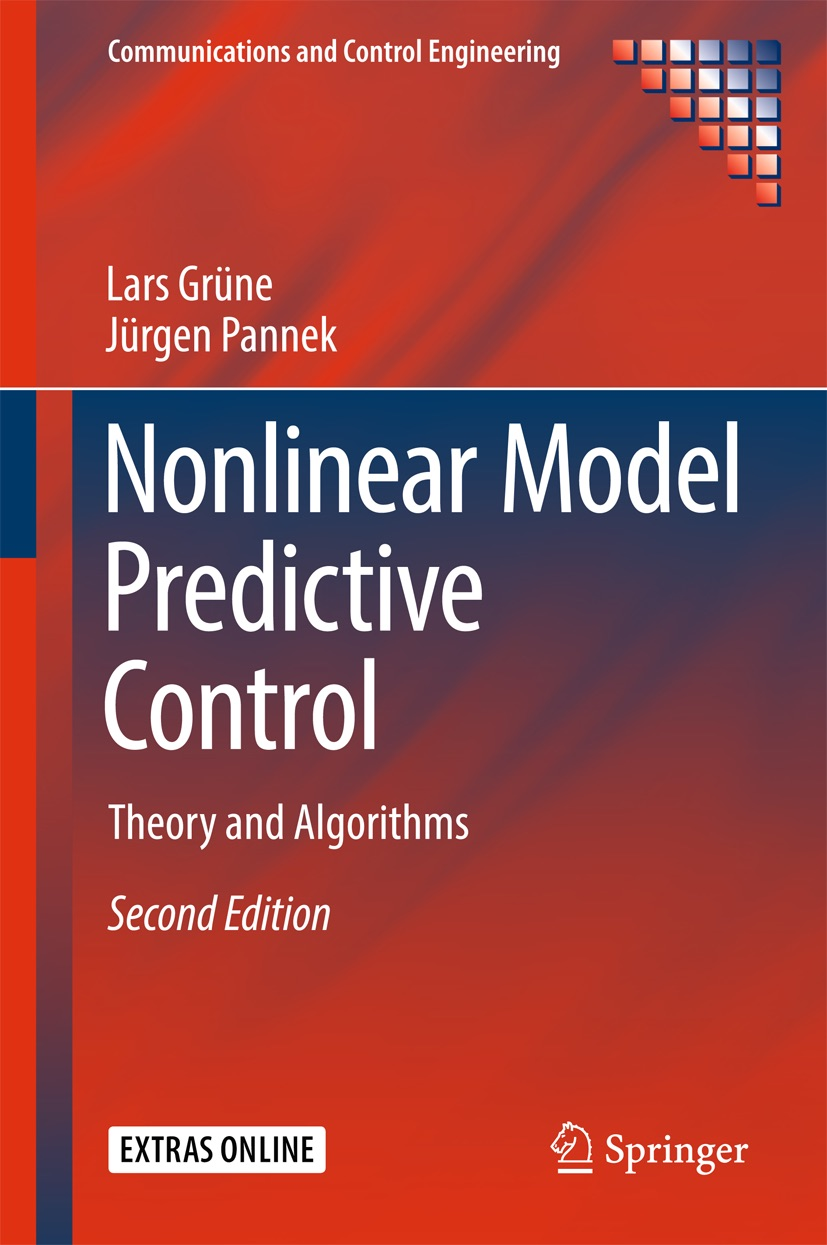## Additional MATLAB and MAPLE routines

On this page we provide MATLAB and MAPLE code which we used for computing several numbers and figures in the book. The MATLAB code should work for MATLAB releases R2007a and newer, the MAPLE code requires MAPLE12 or newer. For more information see Section A.2 in the book and the comments and help texts in each file.

MATLAB routines for solving the Optimization Problem (6.16) ((6.17) in 1st ed.):

For finite time controllability: mpcalpha_cn.m

For exponential controllability: mpcalpha_Csigma.m (needs "mpcalpha_cn.m")

MATLAB routines for plotting the optimal solution of (6.16) ((6.17) in 1st ed.):

Depending on omega: alpha_omega_plot.m (needs "mpcalpha_Csigma.m")

Depending on m: alpha_m_plot.m (needs "mpcalpha_Csigma.m")

MATLAB routines for several numbers and figures:

alpha-values in Example 6.23 (6.20 in 1st ed.): example_6_20.m (needs "mpcalpha_Csigma.m" and "mpcalpha_cn.m")

Figure 10.1 (7.1 in 1st ed.): example_fig_7_1.m (needs "alpha_omega_plot.m")

Figure 10.3 (7.3 in 1st ed.): example_fig_7_3.m (needs "alpha_m_plot.m")

Figure 10.4 (7.4 in 1st ed.): example_fig_7_4.m (caution: very long runtime; plots intermediate results)

MAPLE routines for several numbers, figures and symbolic computations:

Example 5.6: example_5_6.mws

Example 5.8: example_5_8.mws

Example 5.16: example_5_16.mws

Example 5.18: example_5_18.mws

Example 5.19: example_5_19.mws

Example 5.23: example_5_23.mws

Example 6.1: example_6_1.mws

Example 6.2: example_6_2.mws

Example 6.7 (6.5 in 1st ed.): example_6_5.mws

Example 6.23 (6.20 in 1st ed.): example_6_20.mws

Example 7.22 (8.22 in 1st ed.): example_8_22.mws

Example 7.23 (8.23 in 1st ed.): example_8_23.mws

Example 7.30 (8.30 in 1st ed.): example_8_30.mws

Figure 10.5 (7.5 in 1st ed.): example_fig_7_5.mws

Figure 10.6 (7.6 in 1st ed.): example_fig_7_6.mws

ZIP archive with MATLAB routines for solving the optimization problems: matlab_add_solve_opt.zip

ZIP archive with MATLAB routines for plotting the optimal solution: matlab_add_plot_sol.zip

ZIP archive with MATLAB routines for numbers and figures: matlab_add_num_fig.zip

complete ZIP archive with all MATLAB routines: matlab_add_matlab.zip

ZIP archive with MAPLE routines: matlab_add_maple.zip

complete ZIP archive with all MAPLE and MATLAB routines: matlab_add_maple_matlab.zip

→ back to homepage www.nmpc-book.com

© 2010-2016 Lars Grüne, Jürgen Pannek